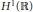Hostname: page-component-797576ffbb-tx785 Total loading time: 0 Render date: 2023-12-05T17:06:19.769Z Has data issue: false Feature Flags: { "corePageComponentGetUserInfoFromSharedSession": true, "coreDisableEcommerce": false, "useRatesEcommerce": true } hasContentIssue false

# The Global Attractor of a Damped, Forced Hirota Equation in H1

Published online by Cambridge University Press:  20 November 2018

## Abstract

Core share and HTML view are not possible as this article does not have html content. However, as you have access to this content, a full PDF is available via the ‘Save PDF’ action button.

The existence of the global attractor of a damped forced Hirota equation in the phase space${{H}^{1}}\left( \mathbb{R} \right)$ is proved. The main idea is to establish the so-called asymptotic compactness property of the solution operator by energy equation approach.

## Keywords

Type
Research Article
Information
Canadian Mathematical Bulletin , 01 June 2010 , pp. 295 - 310

## References

 Abergel, F., Existence and finite dimensionality of the global attractor for evolution equations on unbounded domains. J. Differential Equations 83(1990), no. 1, 85108. doi:10.1016/0022-0396(90)90070-6Google Scholar
 Bourgain, J., Fourier restriction phenomena for certain lattice subsets and applications to nonlinear evolution equations. I. Schrödinger equation. Geom. Funct. Anal. 3(1993), no. 2, 107156. doi:10.1007/BF01896020Google Scholar
 Bourgain, J., Fourier restriction phenomena for certain lattice subsets and applications to nonlinear evolution equations. I. Schrödinger equation. II. The KdV equation. Geom. Funct. Anal. 3(1993), no. 3, 209262. doi:10.1007/BF01895688Google Scholar
 Ghidaglia, J.-M., Weakly damped forced Korteweg-de Vries equations behave as a finite dimensional dynamical system in the long time. J. Differential Equations 74(1988), no. 2, 369390. doi:10.1016/0022-0396(88)90010-1Google Scholar
 Ghidaglia, J.-M., A note on the strong convergence towards attractors of damped forced KdV equations. J. Differential Equations 110(1994), no. 2, 356359. doi:10.1006/jdeq.1994.1071Google Scholar
 Goubet, O., Regularity of the global attractor for a weakly damped nonlinear Schrödinger equation in 2 . Adv. Differential Equations 3(1998), no. 3, 337360.Google Scholar
 Goubet, O., Asymptotic smoothing effect for weakly damped Korteweg-de Vries equations. Discrete Contin. Dynam. Systems, 6(2000), no. 3, 625644.Google Scholar
 Goubet, O. and Rosa, R., Asymptotic smoothing and the global attractor of a weakly damped KdV equation on the real line. J. Differential Equations 185(2002), no. 1, 2553. doi:10.1006/jdeq.2001.4163Google Scholar
 Guo, B. and Tan, S., Global smooth solution for nonlinear evolution equation of Hirota type. Sci. China, Ser. A 35(1992), no. 12, 14251433.Google Scholar
 Hasegawa, A., Optical Solitons in Fibers. Second edition. Springer, Berlin, 1990.Google Scholar
 Hasegawa, A. and Kodama, Y., Nonlinear pulse propagation in a monomode dielectric guide. IEEE J. Quantum Electronics. 23(1987), 510524.Google Scholar
 Huo, Z. and Guo, B., Well-posedness of the Cauchy problem for the Hirota equation in Sobolev spaces Hs . Nonlinear Anal. 60(2005), no. 6, 10931110. doi:10.1016/j.na.2004.10.011Google Scholar
 Huo, Z. and Jia, Y., Well-posedness for the Cauchy problem to the Hirota equation in Sobolev spaces of negative indices. Chinese Ann. Math. Ser. B 26(2005), no. 1, 7588. doi:10.1142/S0252959905000075Google Scholar
 Karachalios, N. and Stavrakakis, N., Global attractor for the weakly damped driven Schrödinger equation in H2R . NoDEA Nonlinear Differential Equations Appl. 9(2002), no. 3, 347360. doi:10.1007/s00030-002-8132-yGoogle Scholar
 Kenig, C. E., Ponce, G., and Vega, L., The Cauchy problem for the Korteweg-de Vries equation in Sobolev spaces of negative indices. Duke Math. J. 71(1993), no. 1, 121. doi:10.1215/S0012-7094-93-07101-3Google Scholar
 Kenig, C. E., Ponce, G., and Vega, L., A bilinear estimate with applications to the Kdv equation. J. Amer. Math. Soc. 9(1996), no. 2, 573603. doi:10.1090/S0894-0347-96-00200-7Google Scholar
 Kodama, Y., Optical solitons in a monomode fiber. J. Statist. Phys. 39(1985), no. 5–6, 597614. doi:10.1007/BF01008354Google Scholar
 Ladyzhenskaya, O., Attractor for Semigroups and Evolution Equations. Lezioni Lincei, Cambridge University Press, 1991.Google Scholar
 Laurey, C., The Cauchy problem for a third order nonlinear Schrödinger equation. Nonlinear Anal. 29(1997), no. 2, 121158. doi:10.1016/S0362-546X(96)00081-8Google Scholar
 Moise, I. and Rosa, R., On the regularity of the global attractor of a weakly damped, forced Korteweg-de Vries equation. Adv. Differential Equations 2(1997), no. 2, 275296.Google Scholar
 Moise, I., Rosa, R., and Wang, X., Attractors for non-compact semigroups via energy equations. Nonlinearity 11(1998), no. 5, 13691393. doi:10.1088/0951-7715/11/5/012Google Scholar
 Rosa, R., The global attractor of a weakly damped, forced Korteweg-de Vries equation in H 1(ℝ) . Mat. Contemp. 19(2000), 129152.Google Scholar
 Tan, S., Han, Y., Long time behavior of solutions to general nonlinear evolution equations. Chinese Ann. Math. Ser. A 16(1995), no. 2, 127141.Google Scholar
 Temam, R., Infinite Dimensional Dynamical Systems in Mechanics and Physics. Second edition. Applied Mathematical Sciences 68, Springer-Verlag, New York, 1997.Google Scholar